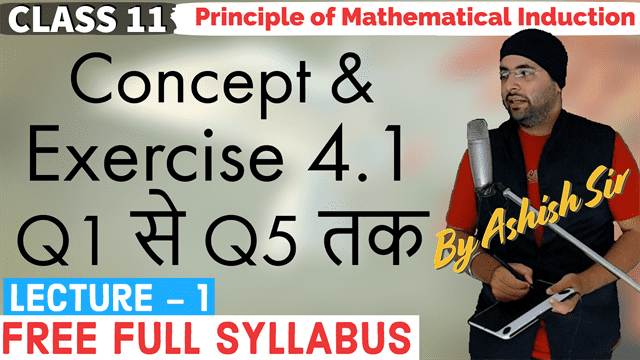# 4. Principle Of Mathematical Induction

Get expert created free online video lectures explaining basics to advance level concepts with detailed pdf notes and assignments for NCERT Chapter 4 Principle of Mathematical Induction Class 11 Maths.

Grow your career and skills at your own pace, with flexible and personalised video lectures designed to build your confidence.

Summary

One key basis for mathematical thinking is deductive reasoning. In contrast to deduction, inductive reasoning depends on working with different cases and developing a conjecture by observing incidences till we have observed each and every case. Thus, in simple language we can say the word ‘induction’ means the generalisation from particular cases or facts.

The principle of mathematical induction is one such tool which can be used to prove a wide variety of mathematical statements. Each such statement is assumed as P(n) associated with positive integer n, for which the correctness for the case n = 1 is examined. Then assuming the truth of P(k) for some positive integer k, the truth of P(k+1) is established.

## Select LectureLecture - 1Lecture - 2Lecture - 3